RPL Palette
The RPL Palette is the dialog from which the operators and functions are selected to build a block or user function. Using the Palette avoids having to type most expressions directly into the Editor, thus limiting the potential for errors.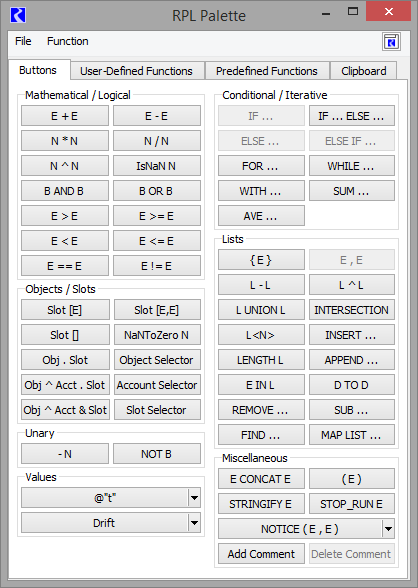The Palette consist of four tabs, one for the buttons, one tab for User-Defined Functions, one tab for the Predefined Functions, and one tab for the Clipboard as follows.
Buttons Tab
On the Palette Buttons tab, each of the buttons represents an operation. The buttons use the following abbreviations:
• B: BOOLEAN
• D: DATETIME
• E: Expression; can be more than one type
• L: LIST
• N: NUMERIC
• Obj: OBJECT
• Slot: SLOT
These buttons represent operations which evaluate to one of the expression data types mentioned above. Buttons on the RPL Palette are enabled and disabled dynamically. When an expression is highlighted in the Editor, the Palette buttons that satisfy the expected data type are enabled. See RPL Palette Buttons for details.
User-Defined Functions Tab
The User-Defined Functions tab shows available user functions in the RPL set or any opened Global Function sets. Select a function for use by double-clicking the function name to replace the selected expression with the function. Figure 3.1 illustrates this tab.
Figure 3.1  User-Defined functions tab of the RPL Palette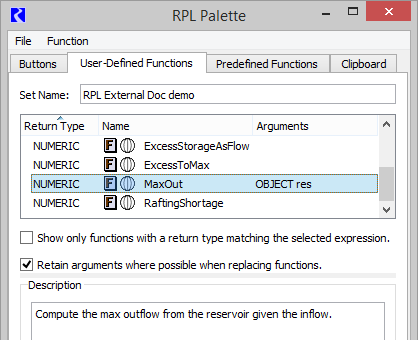There is a toggle to show only functions which have the return type of the selected RPL expression:In addition, there is a toggle to retain the function arguments, when possible, when replacing an existing function.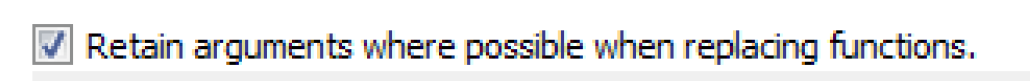When checked, the newly selected function will retain arguments from left to right as long as the types match. If an argument’s type does not match, or if there are not enough existing arguments, the remaining arguments in the new function will remain as empty expressions.
Use the Function, then Show Description menu to show the selected function’s Description as specified on the function’s RPL editor.
Predefined Functions Tab
This tab shows the list of predefined functions available in RiverWare. See About RPL Functions for details. Shown are sortable columns for the Return Type, Name, and the list of Arguments for each function. Figure 3.2 illustrates this tab.
Figure 3.2  Screenshot of the User-Defined functions tab of the RPL Palette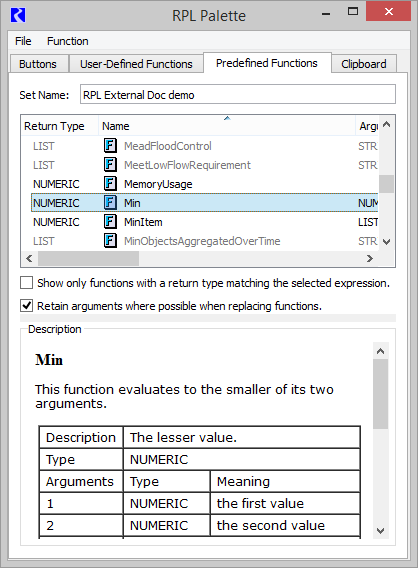There is a toggle to show only functions which have the return type of the selected RPL expression: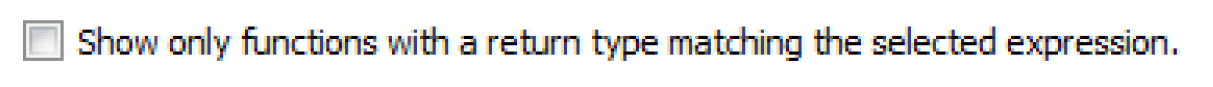When the check box is cleared, all predefined functions are shown even if no expression is selected.
This tab also has a toggle to retain function arguments. as possible, when replacing an existing function. The behavior is the same as described above for the user-defined function tab.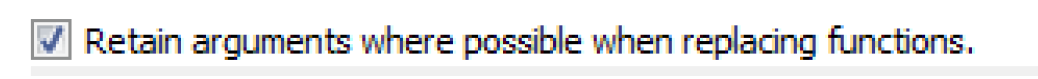Use the Function, then Show Description menu to show the documentation for the selected function. See RPL Predefined Functions for the same documentation.
Note:  References are shown as blue but do not hyperlink elsewhere.
Included is a description, return type, list of the arguments and what they mean, description of evaluation, comments, syntax example and return example.
Clipboard Tab
The clipboard tab is used as a temporary location to hold copied expressions which you can then paste over other expressions. This is particularly useful in editing RPL expressions for readability or performance.
The clipboard maintains a list of the last 100 expressions copied since the workspace was last cleared. When you copy a RPL expression (except from the clipboard itself), a copy of that expression is added to the clipboard as the most recent expression. You can use the Previous and Next buttons to view the entire copy history, and can select and copy an expression or any subexpression in this history. Then, you can paste in other RPL locations to use that expression.
Figure 3.3  Screenshot of the Clipboard tab on the RPL Palette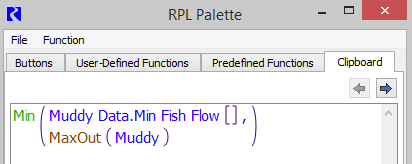The clipboard display is read-only, and supports the following operations:
• Copy (Ctrl+C) - places the selected expression in the copy buffer
• Previous (Alt+Left) - displays the previous copied expression
• Next (Alt+Right) - displays the following copied expression
Note:  The clipboard only tracks copied expressions. Other RPL items, for example, copied statements, function argument declarations, or function constraints are not tracked.
RPL Palette Buttons
This section describes the RPL Palette buttons and their operations.
Mathematical Operation Buttons

Button
Evaluates to
Unspecified Form and Description
E + E
NUMERIC
or
DATETIME
<expr> + <expr>
Addition of two expressions. The two arguments can be numeric or fully specified datetime expressions. If numeric expressions are used, they must be of the same unit type. See Mathematical Expressions Involving a Datetime Operand for details on using this operation with datetimes.
E - E
NUMERIC
or
DATETIME
<expr> - <expr>
Subtraction of two expressions. The two arguments can be numeric or fully specified datetime expressions. If numeric expressions are used, they must be of the same unit type. See Mathematical Expressions Involving a Datetime Operand for details on using this operation with datetimes.
N * N
NUMERIC
<numeric expr> x <numeric expr>
Multiplication of two numeric expressions.
N / N
NUMERIC
<numeric expr> / <numeric expr>
Division of two numeric expressions.
N ^ N
NUMERIC
<numeric expr> ^ <numeric expr>
Exponentiation of one numeric expression (the base) to a power of another numeric expression (the exponent). The exponent is truncated to an integer. (For non-integer exponents, use the Exp RPL Predefined Function; see Exp.) The units of the base are raised to the power of the exponent.
Logical Operation Buttons

Button
Evaluates to
Unspecified Form and Description
IsNaN N
BOOLEAN
IsNaN <numeric expr>
Logical validity check for numeric data types. The result is TRUE if the numeric expression evaluates to a NaN, i.e. Not a Number. The result is FALSE if the numeric expression has a value.
B AND B
BOOLEAN
<boolean expr> AND <boolean expr>
Logical AND comparison of two expressions. The result is TRUE if both expressions are TRUE. The result is FALSE if one expression is TRUE and the other is FALSE.
Note: If the first expression is FALSE, the entire AND is FALSE and the second expression is not evaluated.
B OR B
BOOLEAN
<boolean expr> OR <boolean expr>
Logical OR comparison of two expressions. The result is TRUE if either expression is TRUE. Otherwise it is FALSE.
E > E
BOOLEAN
<expr> > <expr>
Greater than comparison. The result is TRUE if the first expression is strictly greater than the second expression. Otherwise it is FALSE. The two arguments can be numeric expressions of the same unit type or datetime expressions (fully or partially specified). Numeric expressions are automatically converted to common units before comparison. A datetime expression is considered greater if it is later in time.
E >= E
BOOLEAN
<expr> >= <expr>
Greater than or equal comparison. The result is TRUE if the first expression is greater than or equal to the second expression. Otherwise it is FALSE. The two arguments can be numeric expressions of the same unit type or datetime expressions (fully or partially specified). Numeric expressions are automatically converted to common units before comparison. A datetime expression is considered greater if it is later in time.
E < E
BOOLEAN
<expr> < <expr>
Less than comparison. The result is TRUE if the first expression is strictly less than the second expression. Otherwise it is FALSE. The two arguments can be numeric expressions of the same unit type or datetime expressions (fully or partially specified) Numeric expressions are automatically converted to common units before comparison. A datetime expression is considered less than if it is earlier in time.
E <= E
BOOLEAN
<expr> <= <expr>
Less than or equal to comparison. The result is TRUE if the first expression is less than or equal to the second expression. Otherwise it is FALSE. The two arguments can be numeric expressions of the same unit type or datetime expressions (fully or partially specified). Numeric expressions are automatically converted to common units before comparison. A datetime expression is considered less than if it is earlier in time.
E == E
BOOLEAN
<expr> == <expr>
Equality comparison. The result is TRUE if the first expression is exactly equal to the second expression. Otherwise it is FALSE. The two arguments can be numeric expressions (not necessarily of the same unit type), fully or partially specified datetime expressions, object expressions, slot expressions, boolean expressions, string expressions, or list expressions. Numeric expressions of the same unit type are automatically converted to common units before comparison. A fully specified datetime expression can be compared to a partially specified datetime expression; in this case, only the largest time unit is compared.
E != E
BOOLEAN
<expr> != <expr>
Inequality comparison. The result is TRUE if the first expression is not equal to the second expression. Otherwise it is FALSE. The two arguments can be numeric expressions of the same unit type, fully or partially specified datetime expressions, object expressions, slot expressions, boolean expressions, string expressions, and list expressions. Numeric expressions are automatically converted to common units before comparison. Datetime expressions are considered greater as they are later in time. A fully specified datetime expression can be compared to a partially specified datetime expression; in this case, only the largest time unit is compared.
Setting Tolerance for Use in the Logical Comparison Operators
In the above operators, a comparison is made between two values. If the values are numeric, there is the possibility that due to unit conversion or other internal numerical computation, RiverWare could try to compare two numbers that are, in all practical purposes the same, yet are different in the comparison. For example, a RPL expression says (0.0 “cfs” == Flow[]) and the Flow evaluates to 1X10-13 cfs due to the addition of two double precision values. In this example, the statement will return FALSE but probably should return TRUE. To avoid this situation, the user is able to define a tolerance value. From the main RiverWare workspace, select Policy, then RPL Parameters to open the RPL Parameters dialog.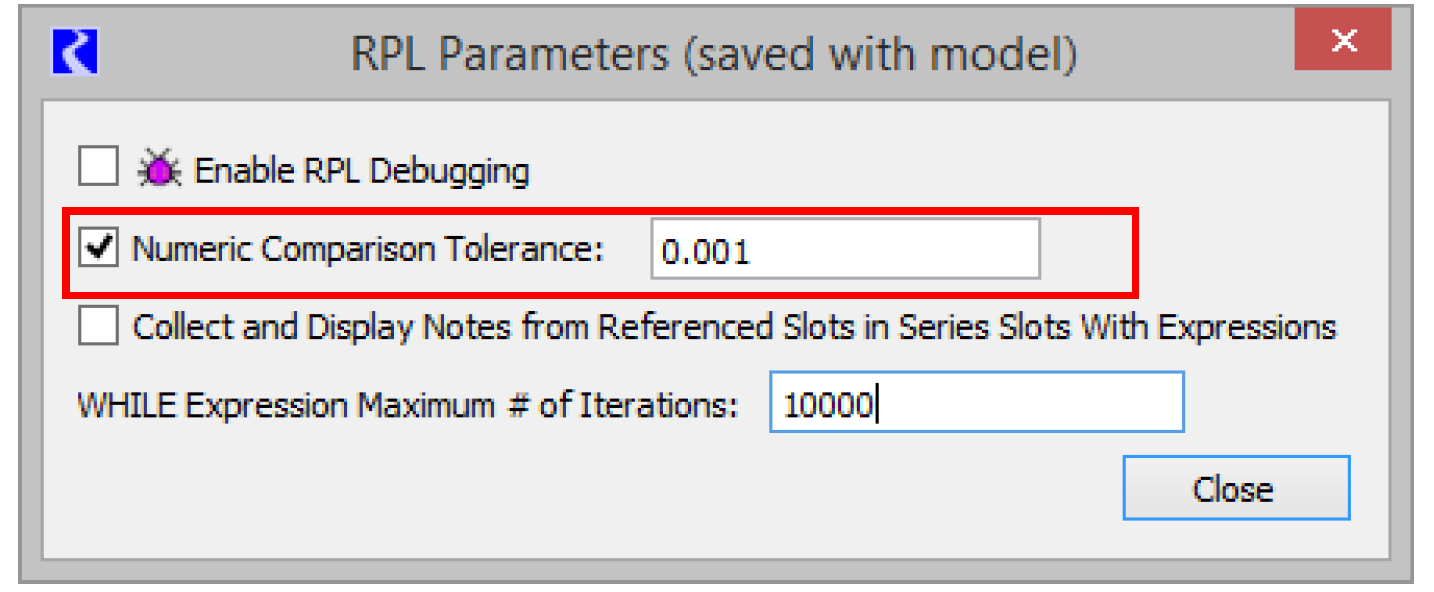Select the Numeric Comparison Tolerance toggle. The box becomes active with a default value of zero. Enter a new value in the box to specify the tolerance. The tolerance represents an absolute value in standard units. Thus, in the example above, if the tolerance is 0.001, it represents that
Abs(0.0 “cfs” - Flow[]) <= 0.001 cms for the comparison to return TRUE. In other words, if two values are within the comparison tolerance of one another, then they are considered equal for the purposes of RPL comparison operations.
Table 3.7 provides details about how the comparison tolerance is applied for each of the RPL comparison operators.

Table 3.7
Operator
Application of Tolerance
RPL Example
(Tolerance = 0.01)
Comparison With Tolerance Applied
Result
E == E
|A - B| <= Tolerance
5.00 cms == 5.00 cms
|5.00 cms - 5.00 cms| <= 0.01 cms
TRUE
5.00 cms == 4.99 cms
|5.00 cms - 4.99 cms| <= 0.01 cms
TRUE
4.98 cms == 5.00 cms
|4.98 cms - 5.00 cms| <= 0.01 cms
FALSE
E != E
|A - B| > Tolerance
5.00 cms != 5.00 cms
|5.00 cms - 5.00 cms| > 0.01 cms
FALSE
5.00 cms != 4.99 cms
|5.00 cms - 4.99 cms| > 0.01 cms
FALSE
4.98 cms != 5.00 cms
|4.98 cms - 5.00 cms| > 0.01 cms
TRUE
E > E
A > B + Tolerance
5.02 cms > 5.00 cms
5.02 cms > 5.01 cms
TRUE
5.01 cms > 5.00 cms
5.01 cms > 5.01 cms
FALSE
5.00 cms > 5.00 cms
5.00 cms > 5.01 cms
FALSE
E >= E
A + Tolerance >= B
5.01 cms >= 5.00 cms
5.02 cms >= 5.00 cms
TRUE
4.99 cms >= 5.00 cms
5.00 cms >= 5.00 cms
TRUE
4.98 cms >= 5.00 cms
4.99 cms >= 5.00 cms
FALSE
E < E
A + Tolerance < B
4.98 cms < 5.00 cms
4.99 cms < 5.00 cms
TRUE
4.99 cms < 5.00 cms
5.00 cms < 5.00 cms
FALSE
5.00 cms < 5.00 cms
5.01 cms < 5.00 cms
FALSE
E <= E
A <= B + Tolerance
4.99 cms <= 5.00 cms
4.99 cms <= 5.01 cms
TRUE
5.00 cms < = 4.99 cms
5.00 cms <= 5.00 cms
TRUE
5.00 cms <= 4.98 cms
5.00 cms <= 4.99 cms
FALSE
The RPL Numeric Comparison Tolerance parameter is saved with the model file and is applied to all RPL sets used by the model including Rulesets, User Defined Accounting Method sets, Optimization Goalsets, Initialization Rules, Iterative MRM sets, Expression Slot sets and Global Functions sets.
Object and Slot Lookup and Assignment Buttons

Button
Evaluates to
Unspecified Form and Description
Slot [ E ]
NUMERIC
<expr> [ <expr> ]
Series slot value at a particular timestep or slot value at a particular row. The first unspecified <expr> must be completed by an expression which evaluates to a specific slot on an object. The second <expr> must be a fully specified datetime which lies on an increment of the model run timestep or a row on the slot.
Slot [ E, E ]
NUMERIC
<expr> [ <expr>, <expr> ]
Table slot value in a particular row and column. The first unspecified <expr> must be completed by an expression which evaluates to a specific table slot on an object. The two comma-separated <expr> are the row and column of the table slot, respectively. The row and column may each be specified as:
A zero-based numeric value with any units.The numeric is rounded down to the nearest integer in those units and the integer is used in the lookup, or
A string expression which matches the column or row label.
OR
Agg series slot or periodic slot value for a particular date and a particular column. The first unspecified <expr> must be completed by an expression that evaluates to a specific slot on an object. The two comma-separated <expr> are the date and column of the slot, respectively. The column may be specified as a zero-based numeric value with units of [NONE] or a string expression which matches the column label. See Referencing Periodic Slots in RPL in User Interface for details.
Slot [ ]
NUMERIC
<expr> [ ]
Series slot value at the current timestep or scalar slot value. The unspecified <expr> must be completed by an expression which evaluates to a specific slot on an object.
NaNToZero N
NUMERIC
NaNToZero <expr>
This operator has a single numeric sub expression; if that sub expression is a lookup of an invalid value (NaN), then NaNToZero evaluates to 0.0 in the units of the lookup slot, otherwise it simply returns the value unchanged. This operator provides a simple and efficient way to treat a missing slot value as zero, a common requirement of water accounting policy.
To illustrate, the following expression
NaNToZero "Res A.Outflow" [ ]
is equivalent to
IF ( IsNaN "Res A.Outflow" [ ] )
0.0 [<Res A.Outflow’s units>]
ELSE
"Res A.Outflow" [ ]
END IF
Note: Unlike the IsNaN operator, this operator is only useful when the sub-expression is an object/slot lookup expression; if any other type of numeric sub expression encounters a NaN during evaluation, then evaluation of the entire containing expression will halt as usual. For example, if we assume that "Res A.Outflow" has units of cms but has no valid values, then the expression
NaNToZero ( "Res A.Outflow" [] ) + 0.0 [cms]
would evaluate to 0.0 [cms], but the expression
NaNToZero ( "Res A.Outflow" [] + 0.0 [cms] )
would not evaluate successfully; it would terminate when the invalid value on Res A was encountered.
Obj . Slot
SLOT
<object expr> . <string expr>
Object and slot expression. This expression can be used to specify the object and slot required by the three slot lookup/assignment expressions above. The object expression must be an object in the model and the string expression must be the name of a slot, exactly as it appears on the object.
Obj ^ Acct . Slot
SLOT
<OBJECT object> ^ <STRING account name> . <STRING slot name>
Object, account and accounting slot expression. This expression can be used to specify the object, account, and slot required by the three slot lookup/assignment expressions. The object expression must be an object in the model. The account is a string expression that returns the account name, for example “Contractor 1” The slot string expression must be the full name of an accounting slot, for example “Storage”.
This ternary operator is preferred in terms of readability and performance over the Obj ^ Acct & Slot operator listed below.
Obj ^ Acct & Slot
SLOT
<object expr> ^ <string expr>
Object and accounting slot expression. This expression can be used to specify the object and slot required by the three slot lookup/assignment expressions above. The object expression must be an object in the model and the string expression must be the full name of an accounting slot, in the form: “account name.slot name”, for example “Contractor 1.Storage”.
This operator was one of the original ways to specify an object, account and slot. Consider using the Obj ^ Acct . Slot ternary operator, described above, for improved performance and readability.
Object Selector
OBJECT
Invokes the object selector dialog.
This button invokes the object selector to choose a single object in the model.
Account Selector
STRING
Invokes the account selector dialog.
This button invokes the account selector to choose a single account as a string.
Slot Selector
SLOT or STRING
Invokes the slot selector dialog.
This button invokes the slot selector to choose a slot. The slot can be on an object or on an account where applicable. In some contexts, the button evaluates to a string which represents the name of a slot, for example “Storage”.
Unary Operation Buttons

Button
Evaluates to
Unspecified Form and Description
- N
NUMERIC
- <numeric expr>
Reverse the sign of a numeric expression. Magnitude and units are maintained, but a positive value becomes negative and a negative value becomes positive.
NOT B
BOOLEAN
NOT <boolean expr>
Reverse the value of a boolean expression. TRUE becomes FALSE and FALSE becomes TRUE.
Values
The Values section is used to access common values and set flags on slots.
Buttons for Common Values
The common values buttons are used to access values that have traditionally been typed by the user. These include common DATETIME values and list expressions.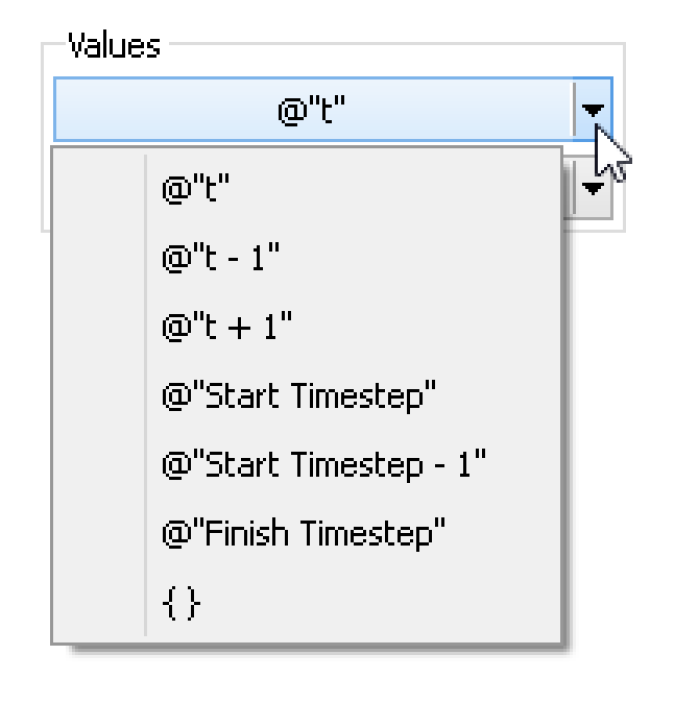• @“t” - Current Timestep
• @“t-1” - Previous Timestep
• @“t+1” - Next Timestep
• @“Start Timestep” - First timestep in the run
• @“Start Timestep - 1” - Initial timestep
• @“Finish Timestep” - Final Timestep in the run period
• { } - Empty list expression
A similar list of common values can be found by right-clicking an expression and choosing the Common Values menu.
Buttons for Setting Flags on Slots
The following RPL operations can only be used to directly set a slot value. They cannot be passed to functions or referenced in block or function logic. They cannot replace any unspecified NUMERIC expression; they can only be used to set a slot.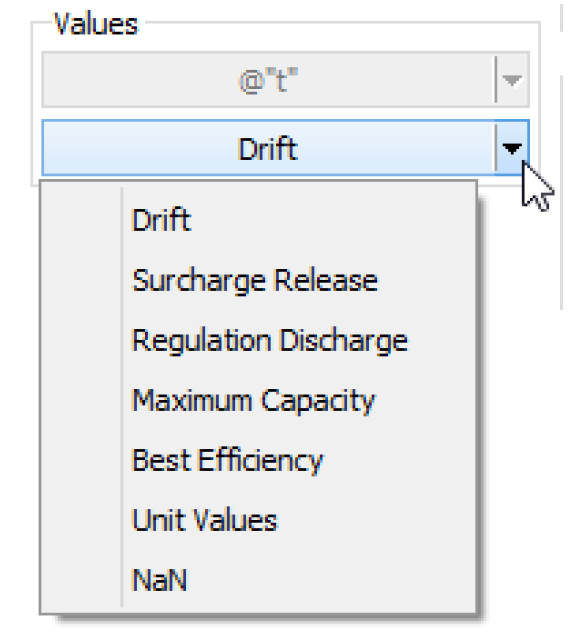Table 3.8
Description
Notes
Drift
Used to set the DRIFT (D) flag on the Regulated Spill or Bypass slots on reservoir objects
The behavior of these operators is identical to the user setting the particular flag through the user interface. The only exception in this case is that the slot can be overwritten by a higher priority rule. When these operators are used, the slot will have both the R flag and the flag associated with the palette button selected. When the object dispatches, the slot will receive the appropriate value. The presence of the these flags will cause the slot to be considered as a known value (for choosing a dispatch method) even though it will not actually have a value until after the object dispatches.
Maximum Capacity
Used to set the MAX CAPACITY (M) flag on the Outflow or Energy slots on reservoir objects.
Surcharge Release
Used to set the SURCHARGE RELEASE (S) flag on the Surcharge Release slot on reservoir objects.
Best Efficiency
Used to set the BEST EFFICIENCY (B) flag on the Energy slot on reservoir objects.
Regulation
Discharge
Used to set the REGULATION_DISCHARGE (G) flag on the Reg Discharge Calculation slot on Control Point objects.
Unit Values
Used to set the UNIT_VALUES (U) flag on Turbine Release or Energy slots on power reservoirs. This flag is used (with the Unit Power Table method) to specify that either Unit Turbine Release or Unit Energy will be specified.
Conditional and Iterative Operations Buttons

Button
Evaluates to
Unspecified Form and Description
IF
any
IF ( <boolean expr> ) THEN
<expr>
END IF
Adds a conditional expression with NO else clause. If the boolean expression evaluates to TRUE, the expression on the second line is evaluated. Otherwise it is not, and the entire conditional statement does not evaluate to a value.
IF ... ELSE
any
IF ( <boolean expr> ) THEN
<expr>
ELSE
<expr>
END IF
Adds a conditional expression including an else clause. If the boolean expression evaluates to TRUE, the expression on the second line is evaluated. If the boolean expression evaluates to FALSE, the expression on the fourth line is evaluated. The entire conditional statement evaluates to one of the two expressions.
ELSE
any
IF ( <boolean expr> ) THEN
<expr>
ELSE
<expr>
END IF
Adds an Else branch to a conditional expression. It is generated by highlighting the either the boolean condition <boolean expr> or consequence expression <expr> of an IF expression or ELSE IF expression and selecting the ELSE button.
If the boolean expression evaluates to FALSE, the expression on the fourth line is evaluated. The entire conditional evaluates to one of the two expressions.
ELSE IF
any
IF ( <boolean expr> ) THEN
<expr>
ELSE IF (<boolean expr>) THEN
<expr>
ELSE IF (<boolean expr>) THEN
<expr>
END IF
Adds an ELSE IF branch to a conditional expression. It is generated by highlighting the boolean condition <boolean expr> or consequence <expr> of an IF or ELSE IF and selecting the ELSE IF button. The ELSE IF branch is added immediately after the branch containing the selection.
In the example above, if the first boolean expression evaluates to FALSE, the boolean expression on the third line is evaluated. If that boolean is true, the expression on the fourth line is evaluated. You may add as many ELSE IF branches as needed.
FOR
any
FOR ( NUMERIC index IN <list expr> ) WITH NUMERIC result = <numeric expr> DO
result = <numeric expr>
END FOR
Structure for looping over the items in a list. The looping variable, index, is set to the value of the next item in the list expression each time through the loop. The value variable, result, is initialized on the first line of the structure. Each time through the loop, the expression on the second line is evaluated and its value is set on the result variable. The previous value of the result variable may be used inside the expression on the second line. The items in the list and the result may be of any expression type even though the default structure uses the numeric type for the looping variable and the result. To change the type of the looping variable, index, and/or the value variable, result, double-click the NUMERIC element and enter the new expression type. The names of the looping and value variables may also be changed.
There is also a FOR statement used to loop over a list and execute multiple statements. See RPL Statements for details.
WITH
any
WITH NUMERIC var = <numeric expr> DO
<expr>
END WITH
Structure for defining a variable to be used multiple times within an expression. The variable, var, is set on the first line of the structure. This variable can be used as many times as desired within the expression(s) between the DO and END WITH. Because the variable is not recalculated each time it is used, this structure can make a block more efficient. The variable may be of any expression type even though the default structure uses the numeric type. To change the type of the variable, var, double-click the NUMERIC element and enter the new expression type. The name of the variable may also be changed.
There is also a WITH statement used to set a temporary variable outside of multiple statements. See RPL Statements for details.
WHILE
any
WHILE ( <boolean expr> ) WITH NUMERIC result = <numeric expr> DO
result = <numeric expr>
END WHILE
Structure for looping as long as a condition is TRUE. The conditional boolean expression is evaluated prior to each loop. If the boolean expression is TRUE, the numeric expression on the second line is evaluated. If the boolean expression is FALSE, the structure stops looping and returns the value of the value variable. The value variable, result, is initialized on the first line of the structure. Each time through the loop, the expression on the second line is evaluated and its value is set on the result variable. The previous value of the result variable may be used inside the expression on the second line. The result may be of any expression type even though the default structure uses the numeric type. To change the type of the value variable, result, double-click the NUMERIC element and enter the new expression type. The name of the value variable may also be changed.
When a WHILE expression is executed, it counts the number of times the body is evaluated, and fails with a message if the iteration count ever exceeds the value of the RPL parameter. The default max iterations is 10,000, but may be modified as needed in the RPL Parameters dialog. (From the main workspace, select Policy, then RPL Parameters to open the parameters).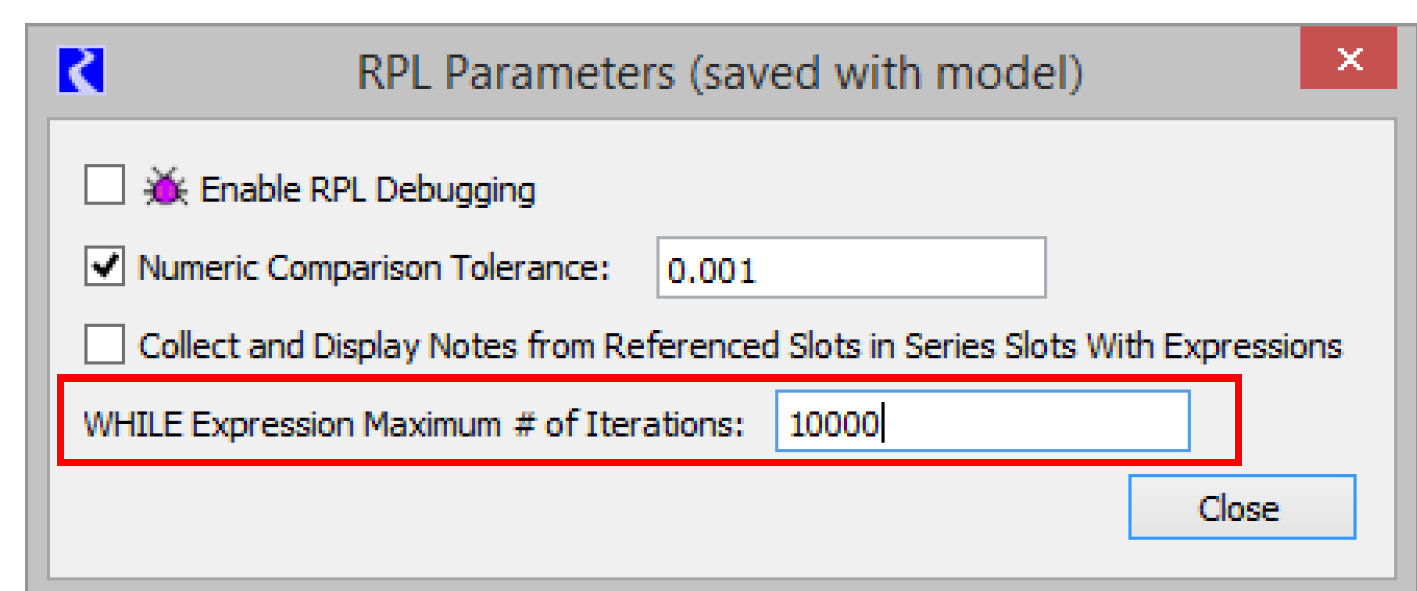SUM
NUMERIC
FOR ( NUMERIC index IN <list expr> ) SUM
<numeric expr>
END FOR
Specialized FOR loop structure for looping over the items in a list and summing a variable. For each item in the list, the looping variable, index, is set to the value of the next item in the list expression. Next, the expression on the second line is evaluated and its value is added to the result variable. The items in the list may be of any expression type even though the default structure uses the numeric type for the looping variable and the result. To change the type of the looping variable, index, double-click the NUMERIC element and enter the new expression type. The names of the looping variables may also be changed.
AVE
NUMERIC
FOR ( NUMERIC index IN <list expr> ) AVE
<numeric expr>
END FOR
Specialized FOR loop structure for looping over the items in a list and averaging their values. For each item in the list, the looping variable, index, is set to the value of the next item in the list expression. Next, the expression on the second line is evaluated and its value is included in the total resulting average. The items in the list may be of any expression type even though the default structure uses the numeric type for the looping variable and the result. To change the type of the looping variable, index, double-click the NUMERIC element and enter the new expression type. The names of the looping variable may also be changed.
List Operation Buttons

Button
Evaluates to
Unspecified Form and Description
{ E }
LIST
{ <expr> }
This button creates a list with a single item of an unspecified type.
E , E
any
<expr>, <expr>
This button adds a new item of an unspecified type to an existing list. To use it, highlight one item and select the button. The new item is added immediately after the highlighted item in the list.
L - L
LIST
<list expr> - <list expr>
The set difference expression takes two lists and returns a single list that contains the items that are in the left hand list but not in the right hand list. Duplicate items are removed.
L ^ L
LIST
<list expr> ^ <list expr>
The set symmetric difference expression takes two lists and returns a single list that contains the items that are not in both lists. This is equivalent to the expression: (list1 U list2) - (list1 intersect list2). This is also equivalent to the expression: (list1 - list2) U (list2 - list1). Duplicate items are removed.
L UNION L
LIST
<list expr> UNION <list expr>
The set union expression takes two lists and returns a single list that contains the items that are in either list or both lists. Duplicate items are removed.
INTERSECTION
LIST
<list expr> INTERSECTION <list expr>
The set intersection expression takes two lists and returns a single list that contains the items that are in both lists. Duplicate items are removed.
L<N>
any
<list expr>< <numeric expr> >
Evaluates to the expression located at the given numeric index in the given list. The index numeric expression is zero-based and has units of [NONE]. If the item at the given index in the list is not the expected type when the expression is evaluated or if there is no item at the given index in the list, the run aborts with an error.
INSERT
LIST
INSERT <expr> INTO <list expr>
Insertion of a new item of unspecified type into the first location of an existing list. If the list is initially empty, the new list contains only one item. If the list initially contains items, all items are shifted down to allow the new item to be inserted at the front of the list.
LENGTH L
NUMERIC
LENGTH <list expr>
Determines the number of items in the list expression. The numeric value has units of [NONE].
APPEND
LIST
APPEND <expr> ONTO <list expr>
Append a new item of unspecified type onto the end of an existing list. If the list is initially empty, the new list contains only one item. If the list initially contains items, the new item is appended as the last item of the list.
E IN L
BOOLEAN
<expr> IN <list expr>
Tests for existence of the given expression as a member of the list expression. If the given expression is one of the items in the list, this function evaluates to TRUE. If the expression does not match any of the items of the list, the function is FALSE.
D TO D
LIST
<datetime expr> TO <datetime expr>
Creates a list of datetimes beginning with the first datetime expression and ending on or before the second datetime expression. The datetime arguments must be fully specified. The interval between datetime items of the list is equal to the run timestep (or the slot’s timestep for an expression slot). If the second datetime argument does not correspond to a model run timestep, the last item of the list is the last model run timestep before the argument.
REMOVE
LIST
REMOVE ITEM @INDEX <numeric expr> FROM <list expr>
Removes the item at a given index from an existing list. All of the items which followed the removed item in the list are shifted up. If there is no item at the given index when the expression is evaluated, the run aborts with an error.
SUB
LIST
SUB <expr> FOR ITEM @INDEX <numeric expr> FROM <list expr>
Substitutes the item at a given index in an existing list with the given expression. If there is no item at the given index when the expression is evaluated, the run aborts with an error.
FIND
NUMERIC
FIND <expr> WITHIN <list expr>
This is used to find the index of a given item in a list. If the item is not contained in the list, -1 (negative one) is returned.
MAP LIST
LIST
MAPLIST (NUMERIC index IN <list expr>) DO
<expr>
END MAPLIST
This expression is used to take a list and perform some action to each element in the list thereby resulting in a new, modified list. This expression was developed primarily for performance reasons. Prior to the existence of this function, the user would use a FOR loop to rotate through the elements of a list, modify them, and create a new list with the modified elements. With the MAP LIST expression, this is much more efficient and more readable.
Miscellaneous Buttons

Button
Evaluates to
Unspecified Form and Description
E CONCAT E
LIST
or
STRING
<expr> CONCAT <expr>
Concatenates two expressions into one. If the two expressions are of type LIST, the resulting expression is a single list containing all of the items of the two original lists. The order of the items is preserved, with the first list’s items before the second list’s items. If the two expressions are of any other type, the resulting expression is a string made up by concatenating the STRINGIFY’d second expression onto the end of the STRINGIFY’d first expression. See the description of STRINGIFY below.
Note: It is not necessary to explicitly STRINGIFY an expression before using it in a CONCAT expression. If the expression is not already a STRING, it will be automatically converted. Thus,
1000 CONCAT “cfs”
is equivalent to
(STRINGIFY 1000) CONCAT “cfs”
but the first expression is cleaner and more efficient
( E )
any
( <expr> )
Adds parenthesis around any expression. Parenthesis can make complicated expressions more readable. Parenthesis may also be required to remove ambiguity in the order of expression evaluation.
STRINGIFY E
STRING
STRINGIFY <expr>
Converts any expression into a string. The STRING representations of other expression types are:
NUMERIC => “number [units]” or “number” if units are {NONE}
DATETIME => “hours:minutes month day, year” or less, depending on model run timestep
BOOLEAN => “TRUE” or “FALSE”
OBJECT => “object name
SLOT => “object name.slot name
LIST => “{ item, item, item ... }”
STOP_RUN E
Aborts the Run
STOP_RUN <expr>
This operator takes any expression type as an argument. When it is evaluated, it aborts the run with an error message which contains the argument as part of the message. If executed from within an iterative MRM rule, it aborts the MRM run.
WARNING(E, E)
NOTICE(E, E)
datatype of the second expression
WARNING(<expr>,<expr>)
NOTICE(<expr>,<expr>)
When one of these expressions is executed, a message is posted to diagnostics for that particular expression (warning, notice, or alert). See Notice, Warning, and Alert Expressions in Debugging and Analysis for more information on these messages.
The first argument of the operator is the content of the diagnostic message and the second argument is returned as the value of the expression.
For example, when the following expression is evaluated, if the estimated flow is negative, a warning is posted to diagnostics and the expression evaluates to the estimated flow, a NUMERIC:
WITH NUMERIC estimatedFlow = EstimateFlow() DO
IF (estimatedFlow < 0.0 cms) THEN
WARNING(“Estimated flow is negative”, estimatedFlow)
ELSE
estimatedFlow
ENDIF
END WITH
comment
<comment>
Inserts a user specified in-line comment above the selected RPL expression or RPL statement. A separate dialog is opened that allows the user to type in a comment. In the RPL editor, the comment is displayed with # characters on the left, lines wrapped as they were in the comment editor dialog, and text in red (or user-specified comment color; see Colors). Double-clicking the comment reopens the edit dialog.
For a given RPL dialog, the inline comments can be hidden or shown using the View, then Show Comments menu or by checking the Show: Comments toggle at the bottom of the dialog.
Delete Comment
Deletes comment
Deletes the selected comment. Comments can also be deleted by selecting the comment, then using the Edit, then Delete menu or pressing Delete.
Units in RPL
In Simulation, all computations are done in internal units, that is cms, m, m3, etc. In RPL, computations are performed in units based on the RPL logic, converting as necessary. For example, if you have an expression 10 cfs + Res.Outflow[ ], the first component will use the value in cfs, the second component will use the value from the slot in cms and then convert to cfs. Finally, it will compute the sum of the two values in cfs.
In terms of units, consider the following:
• You can use any units, even different than slot units.
• When a RPL expression accesses a value on a slot, the slot value is represented internally using a scale of 1.0 and the slots standard units.
• Unit types must always be consistent
• When units are not consistent one value is converted to the other (i.e. 20 cfs + 40 cms)
The unit syntax is “units” with the quotes. But quotes are not necessary unless a “ – ” or “ / ” is used, like “acre-ft”.
Unit Operators
Following are the operators available when specifying RPL units:
• “-” multiplication operator (ex. “acre-ft”)
• “/” division operator (ex. “m/s”)
• “^” raised to the power of". (ex. “m^2”)
• giga = 1e9 (ex. “giga-cfs”)
• mega = 1e6
• kilo = 1000
• pico = 1e-12
The complete list of prefix operators can be viewed in the Units List dialog; see Conversion Factors for RPL Units for details.
Conversion Factors for RPL Units
The conversion factors for all RPL Units can be viewed in the Units List dialog. From the Units menu in the main RiverWare workspace, select List Available Units. Then in the resulting Units List dialog, select the RPL Units tab at the top. At the bottom left of the Units List dialog, there is a check box to Show only units that are present on the workspace. Uncheck the box to show all available units and their conversion factors.
Note:  The RPL Units list only shows the individual components that can be used to construct units in a RPL expression. It does not display separate definitions for units that are combinations of units that are already defined. For example, the flow unit of “acre-ft/day” can be used in a RPL expression, but it is not included in the RPL Units list because it is a combination of an area (acre), length (ft), and time (day) that are already defined. Similarly, “m3” is not listed separately as a volume unit because it is simply a length (m) raised to a power.
Revised: 08/02/2021